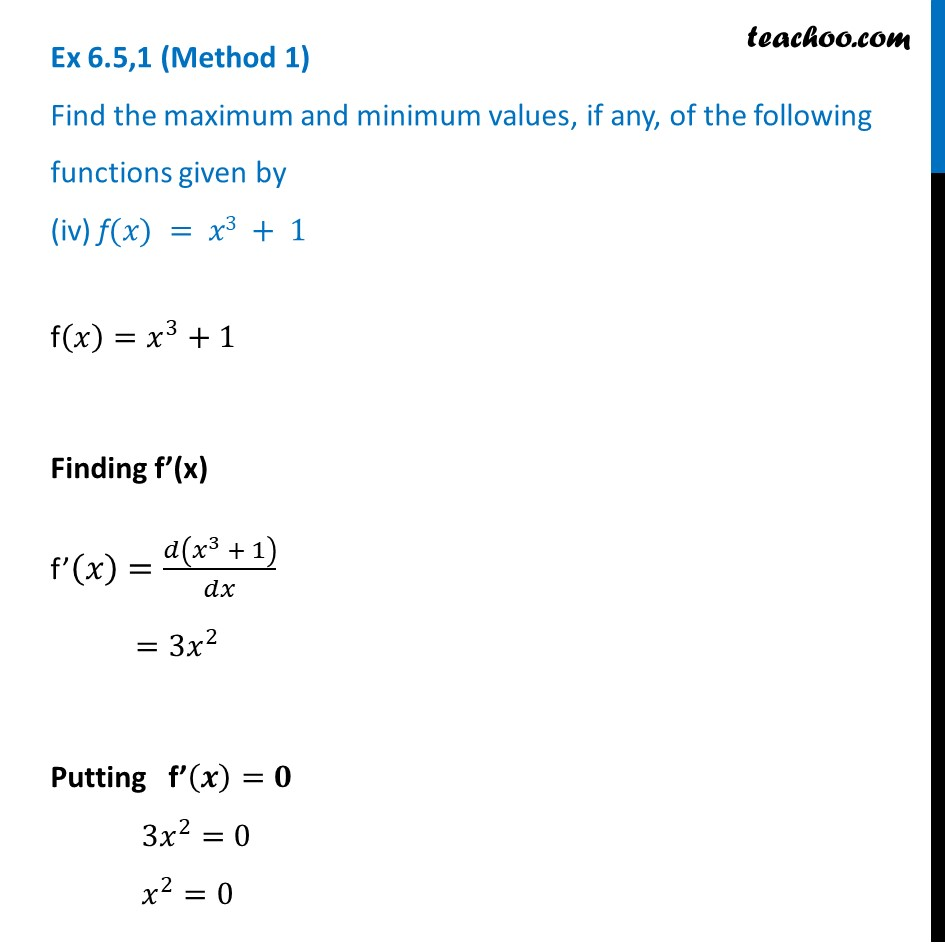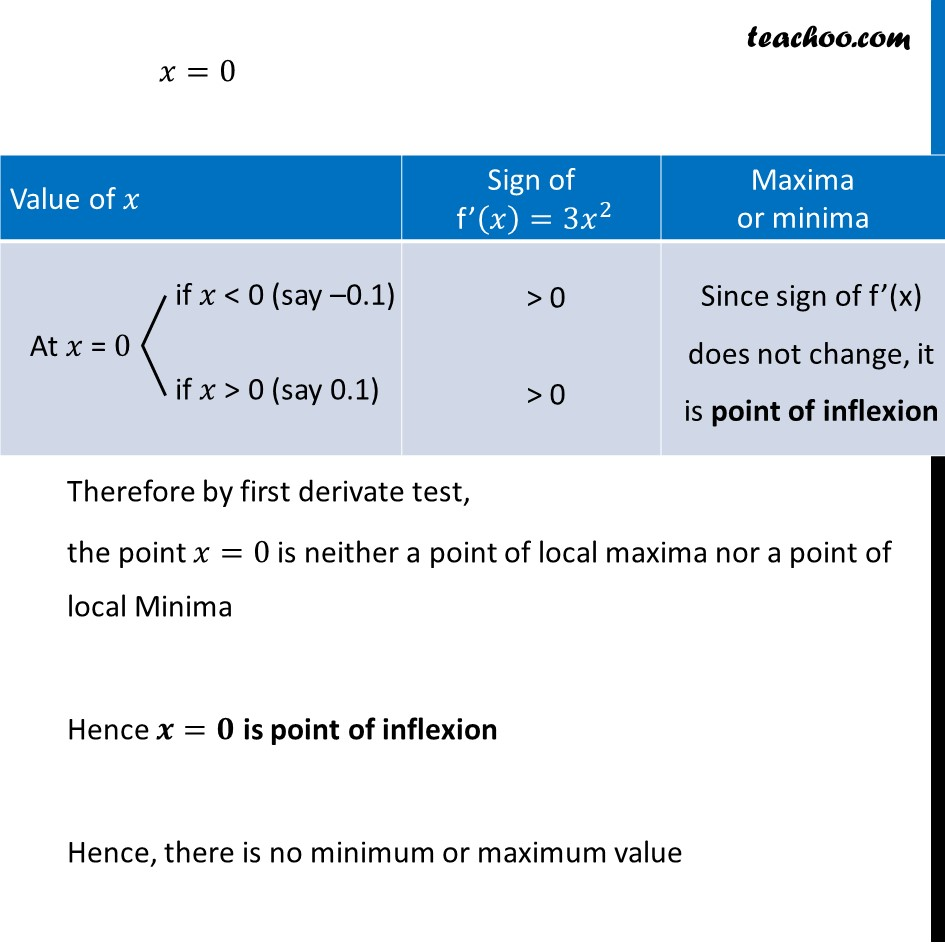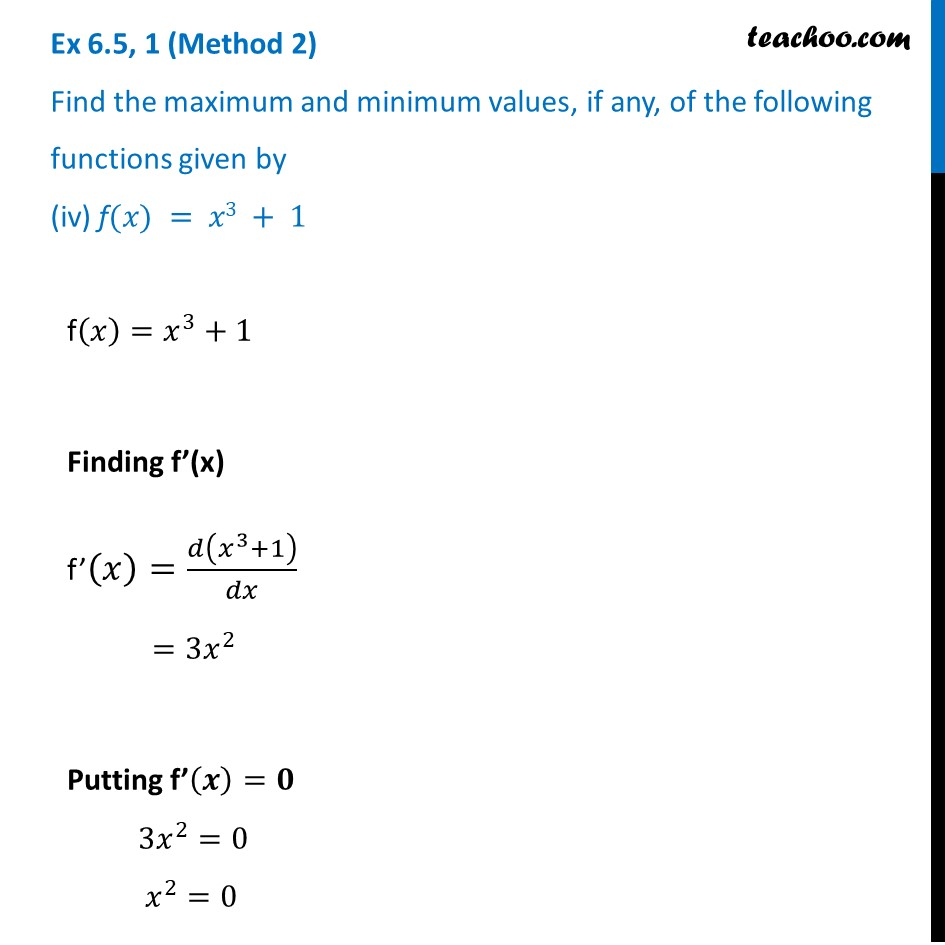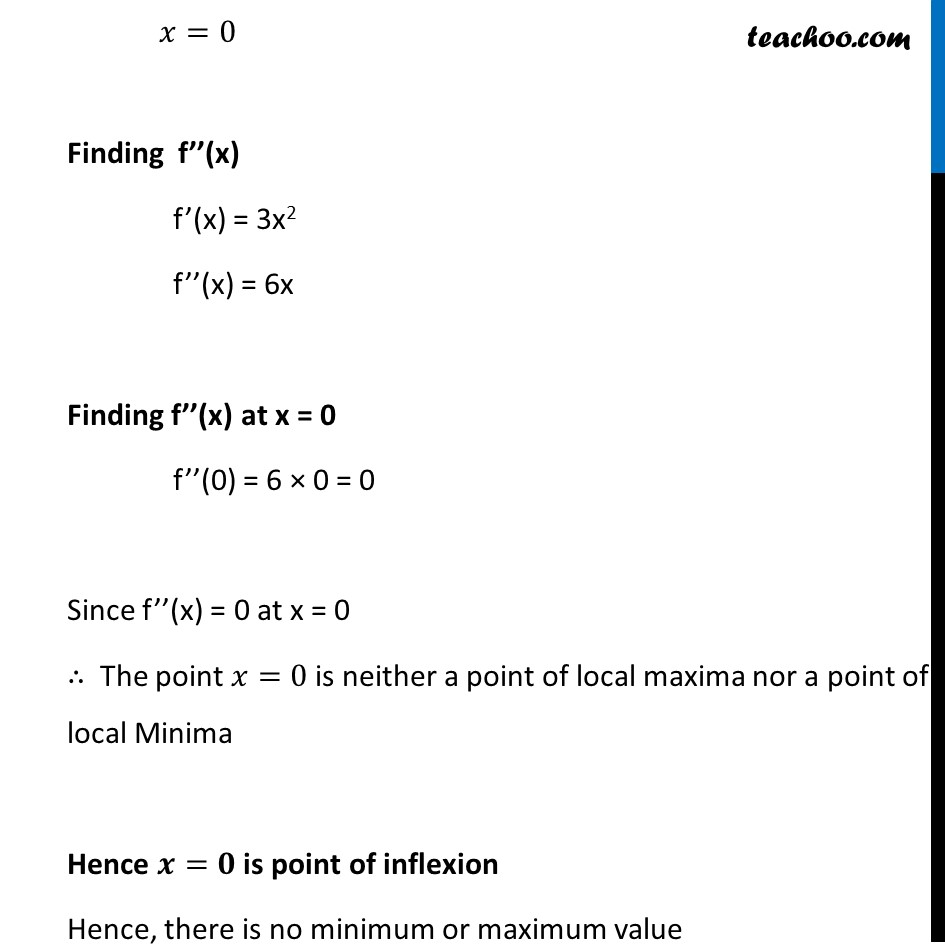Ex 6.3

Chapter 6 Class 12 Application of Derivatives
Serial order wiseLearn in your speed, with individual attention - Teachoo Maths 1-on-1 Class

### Transcript

Ex 6.3,1 (Method 1) Find the maximum and minimum values, if any, of the following functions given by (iv) f(𝑥) = 𝑥3 + 1f(𝑥)=𝑥^3+1 Finding f’(x) f’(𝑥)=𝑑(𝑥^3 + 1)/𝑑𝑥 =3𝑥^2 Putting f’(𝒙)=𝟎 3𝑥^2=0 𝑥^2=0 𝑥=0 Therefore by first derivate test, the point 𝑥=0 is neither a point of local maxima nor a point of local Minima Hence 𝒙=𝟎 is point of inflexion Hence, there is no minimum or maximum value Ex 6.3, 1 (Method 2) Find the maximum and minimum values, if any, of the following functions given by (iv) f(𝑥) = 𝑥3 + 1 𝑥=0 Finding f’’(x) f’(x) = 3x2 f’’(x) = 6x Finding f’’(x) at x = 0 f’’(0) = 6 × 0 = 0 Since f’’(x) = 0 at x = 0 ∴ The point 𝑥=0 is neither a point of local maxima nor a point of local Minima Hence 𝒙=𝟎 is point of inflexion Hence, there is no minimum or maximum value